• 循环置换矩阵
千次阅读
2020-04-08 15:18:41

## 4.5 置换矩阵

是不是任意可逆矩阵都可进行 L D U LDU 分解呢？其实不能，消元操作需要除以对角元素 a i i a_{ii} ，当其为 0 0 时，则会失败。这时可在下面行中选择任一对角元素不为 0 0 的行，对调这两行，则可继续消元。例如

A = [ 0 0 2 1 2 3 0 1 2 ] A= \left[ \begin{matrix} 0 & 0 & 2\\ 1 & 2 & 3\\ 0 & 1 & 2 \end{matrix} \right]

第一行第一个元素 a 11 a_{11} 0 0 ，无法消除第二行第一列的非零元素。矩阵后面两行中，第二行第一个元素 a 21 a_{21} 非零，则对调这两行，矩阵变换为
[ 1 2 3 0 0 2 0 1 2 ] \left[ \begin{matrix} 1 & 2 & 3\\ 0 & 0 & 2\\ 0 & 1 & 2 \end{matrix} \right]

此时第一列元素除对角线外已经都是 0 0 。同理消除第二列时，第二行对角线元素为 0 0 ，此时也需要对调后两行，矩阵变换为

[ 1 2 3 0 1 2 0 0 2 ] \left[ \begin{matrix} 1 & 2 & 3\\ 0 & 1 & 2 \\ 0 & 0 & 2 \end{matrix} \right]

成为上三角阵。

重要性质 对任意可逆矩阵，经过适当的行对调操作，可以分解为 L D U LDU

类似消元操作，行对调操作也可以用矩阵乘法实现，该矩阵称为置换矩阵。

定义 置换矩阵 矩阵 P i j P_{ij} 是单位矩阵 E E 对调 i , j i,j 两行所得。

例如
P 12 = [ 0 1 0 1 0 0 0 0 1 ] P 13 = [ 0 0 1 0 1 0 1 0 0 ] P 23 = [ 1 0 0 0 0 1 0 1 0 ] P_{12}= \left[ \begin{matrix} 0 & 1 & 0\\ 1 & 0 & 0 \\ 0 & 0 & 1 \end{matrix} \right] P_{13}= \left[ \begin{matrix} 0 & 0 & 1 \\ 0 & 1 & 0\\ 1 & 0 & 0 \end{matrix} \right] P_{23}= \left[ \begin{matrix} 1 & 0 & 0 \\ 0 & 0 & 1 \\ 0 & 1 & 0 \end{matrix} \right]

矩阵 A A 列向量左乘置换矩阵 P i j P_{ij} 就是对调向量的 i , j i,j 两个分量。
P i j a k = P i j ( a 1 k , ⋯   , a i k , ⋯   , a j k , ⋯   , a m k ) = ( a 1 k , ⋯   , a j k , ⋯   , a i k , ⋯   , a m k ) P_{ij}\mathbf{a}_k = P_{ij}(a_{1k},\cdots,a_{ik},\cdots,a_{jk},\cdots,a_{mk}) = (a_{1k},\cdots,a_{jk},\cdots,a_{ik},\cdots,a_{mk})

P i j A P_{ij}A 就是对调矩阵 A A ( i , j ) (i,j) 两行。

置换矩阵是正交矩阵， P T P = E P^TP=E 。对矩阵进行多次行对调操作，就是多个置换矩阵连乘，记为 P P P P 是单位矩阵 E E 进行相应的多次行对调结果。

P = P 21 P 32 = [ 0 0 1 1 0 0 0 1 0 ] P=P_{21}P_{32}= \left[ \begin{matrix} 0 & 0 & 1\\ 1 & 0 & 0 \\ 0 & 1 & 0 \end{matrix} \right]

重要性质 对任意可逆矩阵 A A ，经过适当的行对调操作 P P ，可以分解为 P A = L D U PA = LDU

我们还可以换个角度看待 P A = L D U PA = LDU ，由于各矩阵均可逆，得 ( L D U ) − 1 P A = E (LDU)^{-1}PA = E ，令 P ′ = ( L D U ) − 1 P P'=(LDU)^{-1}P P ′ A = E P'A=E ，这说明 P ′ P' 是逆矩阵 A − 1 A^{-1} 。通过高斯消元法可得到逆矩阵 A − 1 = U − 1 D − 1 L − 1 P A^{-1}=U^{-1}D^{-1}L^{-1}P ，对角阵 D D 可逆，需对角元素均不为零，故矩阵 A A 主元均不为零时，矩阵 A A 可逆。

当矩阵 A A 是对称矩阵时，假设没有行对调，则 S = L D U S = LDU ，取转置， S T = ( L D U ) T = U T D T L T = U T D L T = S = L D U S^T = (LDU)^T=U^TD^TL^T=U^TDL^T=S=LDU ，所以有 L T = U L^T=U 成立。

重要性质 对称矩阵，假设没有行对调，则可以分解为 S = L D L T S = LDL^T

更多相关内容
• 有效地计算基本 NxN 循环置换矩阵的 'l' 次幂（默认值：l = 1）。 基于“循环置换矩阵”的定义：“矩阵分析”，Rogers A. Horn 和 Charles A. Johnson，剑桥大学出版社，1985（第 26 页） 例如 rand*circperm(5,1...matlab
• 基于AWGN信道循环置换矩阵的LDPC编码的新构造研究论文
•研究论文
• 借助于快速傅氏变换(FFT)技术，给出了计算2个n阶置换因子循环矩阵之乘积阵的一种快速算法，其算术复杂性为O(nlog2n)，最后给出一个算例。
• PMn(B)表示布尔代数B = ｛0,1｝上的所有nxn置换因子循环矩阵组成的集合．PMn(B)对于矩阵乘法成为一个半群．刻画了PMn(B)中的幂等元,并给出了半群PMn(B)中的Euler-Fermat定理．
• ## 置换矩阵分解

千次阅读 2018-10-13 20:09:43
从Eigen 3.3开始，LU，Cholesky和QR分解可以在适当的位置运行，即直接在给定的输入矩阵内运行。 在处理大型矩阵时，或者当可用内存非常有限时（嵌入式系统），此功能特别有用。为此，必须使用Ref &lt;&gt;...

从Eigen 3.3开始，LU，Cholesky和QR分解可以在适当的位置运行，即直接在给定的输入矩阵内运行。 在处理大型矩阵时，或者当可用内存非常有限时（嵌入式系统），此功能特别有用。为此，必须使用Ref <>矩阵类型实例化相应的分解类，并且必须使用输入矩阵作为参数来构造分解对象。 作为一个例子，让我们考虑使用部分旋转的就地LU分解。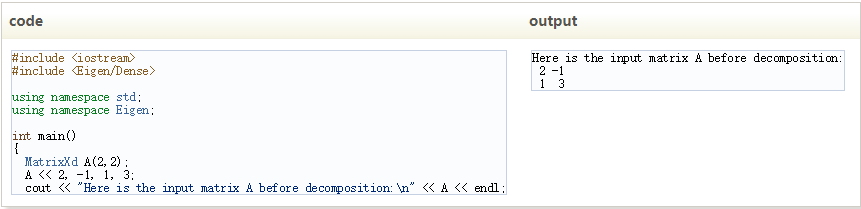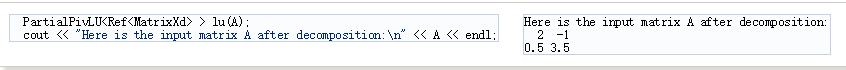这里，lu对象计算并存储由矩阵A保持的存储器中的L和U因子。因此，A的系数在因子分解期间被破坏，并且由L和U因子代替，因为可以验证：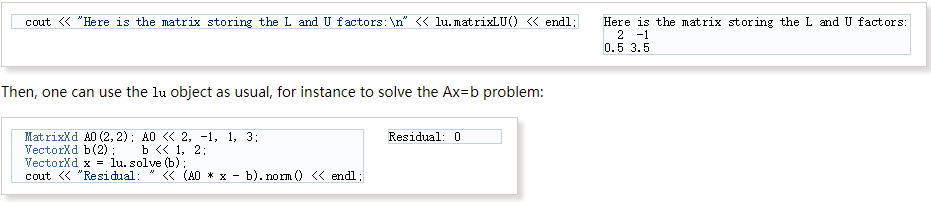由于存储器在A和lu之间共享，因此修改矩阵A将使lu无效。 通过修改A的内容并尝试再次解决初始问题，可以轻松验证这一点：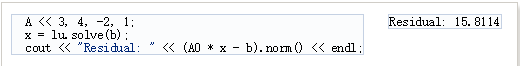请注意，调用compute不会更改lu对象引用的内存。 因此，如果使用不同于A的另一个矩阵A1调用计算方法，则不会修改A1的内容。 这仍然是将用于存储矩阵A1的L和U因子的A的内容。 这可以很容易地验证如下：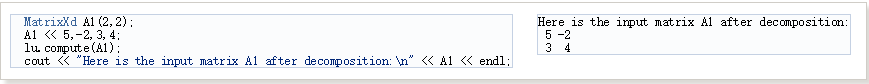矩阵A1不变，因此可以求解A1 * x = b，并直接检查残差而不需要A1的任何副本：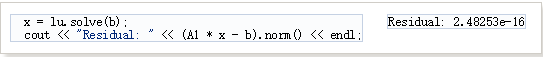展开全文• 置换矩阵 题解 首先对于有大于1个环的情况，明显行列式值是为零的。 因为这种情况必定有一个环的长度小于∣n2∣\left|\frac{n}{2}\right|∣∣​2n​∣∣​，所以就一定可以将一个环的区域全部消成0，这样答案就肯定...

# 置换矩阵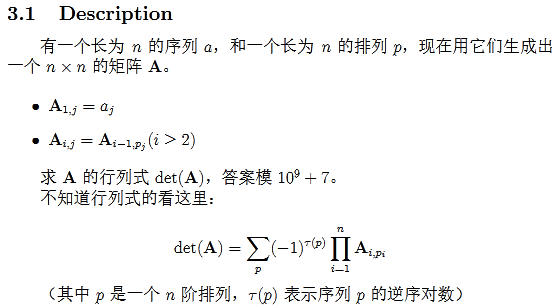## 题解

首先对于有大于1个环的情况，明显行列式值是为零的。
因为这种情况必定有一个环的长度小于 ∣ n 2 ∣ \left|\frac{n}{2}\right| ，所以就一定可以将一个环的区域全部消成0，这样答案就肯定为0了。

那么对于 p 1 = n , p i = i − 1 p_{1}=n,p_{i}=i-1 的部分呢，我可以很容易看出这是一个循环行列式。
对于循环行列式的值，我们有着一种特别的求法。
d e t ( A ) = ∏ i = 0 i − 1 ( ∑ j = 0 n − 1 a j ω i j ) det(A)=\prod_{i=0}^{i-1}(\sum_{j=0}^{n-1}a_j\omega^{ij})
f ( x ) = ∑ j = 0 n − 1 a j x j f(x)=\sum_{j=0}^{n-1}a_jx^{j} ，那么有
d e t ( A ) = ∏ i = 0 i − 1 f ( ω i ) det(A)=\prod_{i=0}^{i-1}f(\omega^i)
其中 ω \omega 表示单位根

证明
考虑范蒙德矩阵，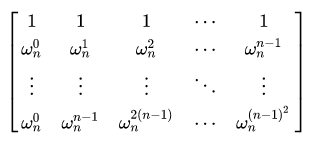其中 ω n \omega_n 表示 n n 次单位根，即 ω n = e 2 π n \omega_{n}=e^{\frac{2\pi}{n}}
将范德蒙矩阵与 n n 阶循环行列式相乘，由于 ω n n + k = ω n k \omega_n^{n+k}=\omega_n^k ，有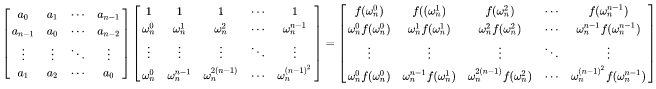两边取行列式，则有
d e t ( A ) d e t ( V ) = ( ∏ i = 0 n − 1 f ( ω n j ) ) d e t ( V ) det(A)det(V)=(\prod_{i=0}^{n-1}f(\omega_n^j))det(V)
考虑到 d e t ( V ) = ∏ 0 ⩽ j < i < n ( ω n i − ω n j ) det(V)=\prod_{0\leqslant j<i<n}(\omega_n^i-\omega_n^j)
w n 0 , w n 1 , . . . w n n − 1 w_n^0,w_n^1,...w_n^{n-1} 互不相等，故 d e t ( V ) ≠ 0 det(V)\not = 0 ，有
d e t ( A ) = ∏ i = 0 i − 1 f ( ω i ) det(A)=\prod_{i=0}^{i-1}f(\omega^i)
证毕

但我们在模的意义下有如何计算呢？
考虑到 ω 0 , ω 1 , . . . , ω n − 1 \omega^0,\omega^1,...,\omega^{n-1} B ( x ) = x n − 1 B(x)=x^n-1 的所有根，我们可以通过这东西来进行计算。
λ 1 , λ n \lambda_1,\lambda_n n n 次多项式 A ( x ) = f ( x ) A(x)=f(x) 的所有根， μ 1 , . . . , μ m \mu_1,...,\mu_m m m 次多项式 B ( x ) B(x) 的所有根，
定义函数 F ( A ， B ) = b m n ∏ i = 1 m A ( μ i ) F(A，B)=b_m^n\prod_{i=1}^{m}A(\mu_i) ，显然 F ( A , B ) F(A,B) 就是我们要求的答案
显然有
F ( A , B ) = b m n ∏ i = 1 n A ( μ i ) = a n m b m n ∏ ( μ i − λ j ) = ( − 1 ) n m a n m ∏ i = 1 n B ( λ i ) F(A,B)=b_m^n\prod_{i=1}^{n}A(\mu_i)=a_n^mb_m^n\prod(\mu_i-\lambda_j)=(-1)^{nm}a_n^m\prod_{i=1}^{n}B(\lambda_i)
结合定义，可以得到以下性质

• F ( A , B ) = ( − 1 ) n m F ( B , A ) F(A,B)=(-1)^{nm}F(B,A)
• F ( A , B ) = a n m b m n ( n = 0 ∨ m = 0 ) F(A,B)=a_n^mb_m^n(n=0\lor m=0)
• F ( A − C B , B ) = F ( A , B ) ( b m = 1 ) F(A-CB,B)=F(A,B)(b_m=1)
• F ( A , B ) = ( − 1 ) d e g ( A ) − d e g ( A − C B ) F ( A − C B , B ) F(A,B)=(-1)^{deg(A)-deg(A-CB)}F(A-CB,B)

于是此时我们就可以 O ( n 2 ) O(n^2) 地计算出这样行列式的值了。

但对于不是循环行列式的行列式呢？
我们发现我们对于原循环求出的行列式可以通过对某些列的交换达到来改变原序列，从而使它成为循环行列式。
我们接着就可以发现交换次数只会改变的行列式的正负，而交换次数又是等于点一的路径的序列的逆序对数的。

总时间复杂度还是 O ( n 2 ) O\left(n^2\right)

## 源码

一个正负判断了一个下午。。。

#include<cstdio>
#include<cmath>
#include<cstring>
#include<iostream>
#include<algorithm>
#include<vector>
#include<queue>
#include<set>
#include<map>
using namespace std;
#define MAXN 5005
#define lowbit(x) (x&-x)
#define reg register
typedef long long LL;
typedef unsigned long long uLL;
typedef unsigned int uint;
typedef pair<int,int> pii;
const int INF=0x7f7f7f7f;
const int mo=1e9+7;
const double PI=acos(-1.0);
template<typename _T>
_T Fabs(_T x){return x<0?-x:x;}
template<typename _T>
_T f=1;x=0;char s=getchar();
while(s>'9'||s<'0'){if(s=='-')f=-1;s=getchar();}
while('0'<=s&&s<='9'){x=(x<<3)+(x<<1)+(s^48);s=getchar();}
x*=f;
}
int n,m,a[MAXN],p[MAXN],q[MAXN],A[MAXN],B[MAXN],pp[MAXN];
int qkpow(int a,int s){int t=1;while(s){if(s&1)t=1ll*a*t%mo;a=1ll*a*a%mo;s>>=1;}return t;}
signed main(){
freopen("matrix.in","r",stdin);
freopen("matrix.out","w",stdout);
now=q;tmp++;while(now^1)pp[tmp+1]=now,now=q[now],tmp++;
if(tmp<n){puts("0");return 0;}
for(int i=1;i<=n;i++)for(int j=i+1;j<=n;j++)if(pp[i]>pp[j])sum++;
for(int i=0;i<n;i++)A[i]=a[pp[i+1]];B=mo-1;B[m]=1;n--;ans=1;
while(1){
if(n<m)swap(A,B),ans=(n&m)&1?mo-ans:ans,swap(n,m);
if(!m){ans=1ll*ans*qkpow(B,n)%mo;break;}const int d=1ll*A[n]*qkpow(B[m],mo-2)%mo;
int L=0;while(n&&!A[n])n--,L++;ans=1ll*ans*qkpow(B[m],L)%mo;
}
return 0;
}


## 谢谢！！！

展开全文• ## ACM-矩阵之置换

千次阅读 2014-10-10 00:47:11
ACM-矩阵置换

还有一类数学题目，那就是置换，其本质就是一一映射，具体参看训练指南144页。可能正如同书中所说的，我们一般处理置换时常常将它们写成循环乘积的形式，这样便于理解和计算。可是如果当我们进行的置换的次数非常多，那么循环乘积可能就会变得比较繁杂，即使任然可以计算，但是在时间上也是不允许的。那么有没有什么方法可以加速置换运算呢？那就是矩阵。首先想这样一个事实，任何置换都可以写成矩阵的形式，如图所示：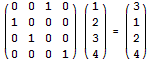上图利用矩阵将{1；2；3；4}置换成了{3；1；2；4}，的确是这样的，只要变换第一个矩阵，那么乘上第二个矩阵后的结果就会随着改变，如果我们精心构造，那么有一定会出现我们想要的结果。到这里，方法就很明显了，类是于矩阵求解递推式，我们将第一个矩阵开n次幂，代表数据被置换了n次，然后乘上初始矩阵（原始数据），记为A^n * B = C，最终得到的矩阵里就会出现原始数据被置换了n次之后的数据，那就是我们想要的答案，时间复杂度上依然使用快速幂算法优化，至于如何构造矩阵，这是由题目给出的置换矩阵决定的。下面我们就具体看一道例题，来掌握矩阵求解置换的思想，HDOJ：2371，时空转移（点击打开链接），题目如下：

# Decode the Strings

Time Limit: 1000/1000 MS (Java/Others)    Memory Limit: 32768/32768 K (Java/Others)
Total Submission(s): 768    Accepted Submission(s): 233

Problem Description
Bruce Force has had an interesting idea how to encode strings. The following is the description of how the encoding is done:

Let x 1,x 2,...,x n be the sequence of characters of the string to be encoded.

1. Choose an integer m and n pairwise distinct numbers p 1,p 2,...,p n from the set {1, 2, ..., n} (a permutation of the numbers 1 to n).
2. Repeat the following step m times.
3. For 1 ≤ i ≤ n set y i to x pi, and then for 1 ≤ i ≤ n replace x i by y i

For example, when we want to encode the string "hello", and we choose the value m = 3 and the permutation 2, 3, 1, 5, 4, the data would be encoded in 3 steps: "hello" -> "elhol" -> "lhelo" -> "helol".

Bruce gives you the encoded strings, and the numbers m and p 1, ..., p n used to encode these strings. He claims that because he used huge numbers m for encoding, you will need a lot of time to decode the strings. Can you disprove this claim by quickly decoding the strings?

Input
The input contains several test cases. Each test case starts with a line containing two numbers n and m (1 ≤ n ≤ 80, 1 ≤ m ≤ 10 9). The following line consists of n pairwise different numbers p 1,...,p n (1 ≤ p i ≤ n). The third line of each test case consists of exactly n characters, and represent the encoded string. The last test case is followed by a line containing two zeros.

Output
For each test case, print one line with the decoded string.

Sample Input

5 3
2 3 1 5 4
helol
16 804289384
13 10 2 7 8 1 16 12 15 6 5 14 3 4 11 9
scssoet tcaede n
8 12
5 3 4 2 1 8 6 7
encoded?
0 0


Sample Output

hello
second test case
encoded?


题意：
给出n个数字，代表一次置换，数字本身是下标的意思，如置换：2 3 1 5 4，代表：将第一个位置上的换成第二个位置上的，将第二个位置上的换成第三个位置上的，将第三个位置上的换成第一个位置上的，将第四个位置上的换成第五个位置上的，将第五个位置上的换成第四个位置上的，但是对于这道题来说，置换的对象是一串字符，自然操作就针对的是每一个字符了，好了，理解了置换，后面就轻松了。题目最后的要求就是在给定置换次数m和经过置换后的结果，求出最初的字符串。

分析：
题目会给出置换的次数m，和字符串经过置换后的最终结果，这里稍微有些不同，题目要求的是通过结果求最初始的字符串。先来想想正常情况，我们可以通过矩阵计算置换，即：A ^m * B = C，A为构造矩阵，B为初始矩阵，这里C是未知的，需要计算的，而在本题中已知的是C，要求的是B，其实变换一下就行：B = C * A^(-m) = (A的逆)^m * C 。我们先来构造矩阵A，首先假定初始矩阵B = {1；2；3；4；5}，然后我们先来看第一个测试用例，它给出的转置矩阵为{2；3；1；5；4}，其实它就是矩阵C，因为在一次转置运算中，转置矩阵就代表着答案本身，由此我们便可以构造出A了，详见下图：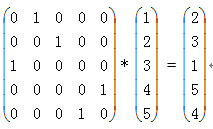可能大家就说了，这只是第一个测试用例的构造矩阵呀，的确是的，不同的转置矩阵对应着不同的构造矩阵，那怎么办呢？其实A矩阵是有规律的，不难发现A矩阵中只有0或1，如果我们统一B矩阵为{1；2；3；4；5}的话，那么要使上图中的等式成立，当且仅当A矩阵相对于置换矩阵C的对应行中的对应列为1，其余为0，比如用例一的C为{2；3；1；5；4}，那么对应构造矩阵A的第1行第2列为1，第2行第3列为1，第3行第1列为1，第4行第5列为1，第5行第4列为1，所以我们可以利用题目给出的置换矩阵来构造出A，至于A的逆，因为在这里A是特殊的，它是正交矩阵，所以它的逆等于它的转置。如此一来，用快速幂解之即可。

源代码：
#include <cstdio>
#include <cstring>
#include <algorithm>
using namespace std;

const int MAXN = 100;
const int MOD  = 1e5;
struct Mat
{
int n, m;
int mat[MAXN][MAXN];
Mat()
{
memset(mat, 0, sizeof(mat));
n = m = MAXN;
};
void init()                                       // 初始化为单位矩阵
{
for(int i=1; i<=n; ++i)
for(int j=1; j<=m; ++j)
mat[i][j] = (i==j);
}
Mat operator * (Mat b)                             // 重载乘法
{
Mat c;
c = Mat();
c.n = n;
c.m = b.m;
for(int i=1; i<=n; ++i)                        // 注意这里从1开始
for(int j=1; j<=b.m; ++j)
{
//if(mat[j][i] <= 0)  continue;        // 剪枝
for(int k=1; k<=m; ++k)
{
//if(b.mat[i][k] <= 0) continue;   // 剪枝
c.mat[i][j] += (mat[i][k]*b.mat[k][j]); //% MOD;
//c.mat[i][j] %= MOD;
}
}
return c;
}
Mat QuickPow(Mat a, int k)                         // 快速幂
{
Mat c;
c.n = a.n;
c.m = a.n;
c.init();                                      // 初始化为单位矩阵
while(k)
{
if(k & 1)
c = c*a;
a = a*a;
k >>= 1;
}

return c;
}
Mat transpose()                                    // 求矩阵的转置
{
Mat c;
c.n = m;
c.m = n;
for(int i=1; i<=n; ++i)
for(int j=1; j<=m; ++j)
c.mat[j][i] = mat[i][j];
return c;
}
};

int main()
{//freopen("sample.txt", "r", stdin);
int n, m;
char ch;

while(~scanf("%d%d", &n, &m) && (n||m))
{
Mat ini, base, ans;
base.n = base.m = n;
for(int i=1; i<=n; ++i)                       // 初始矩阵
base.mat[i] = i;
ini.n = ini.m = n;
for(int i=1; i<=n; ++i)                       // 构造矩阵
{
int a;
scanf("%d", &a);
ini.mat[i][a] = 1;
}
getchar();
gets(ch+1);
ini = ini.transpose();                        // 取转置
ini = ini.QuickPow(ini, m);                   // 求幂次
ans = ini * base;
for(int i=1; i<=n; ++i)
printf("%c", ch[ans.mat[i]]);          // 答案为第一列
puts("");
}
return 0;
}


需要说明的是，在这道题目中，虽然中间结果不会很大，但是我为了保险，在最开始的时候还是取了模，但结果是超时，去掉模运算后就过了，说明模还是比较费时的，需慎用。其它类似的题目还有，VOJ：1049。
接下来，我们将讨论矩阵运算的最后一个部分，即仿射变换，同时还将给出有关矩阵的一份比较全面的模版，传送门（ 点击打开链接）。

展开全文acm
• 本代码只支持24位明文进行加密或是解密，设置循环可持续加密/解密，加密与解密分别是两个不同的子函数，代码如下： #include <stdio.h> #include <string.h> #define N 24 void encrypt(); void ...c语言
• ## 循环矩阵

千次阅读 2016-12-05 22:46:06
今天讲讲线性代数中的一类特殊的矩阵———循环矩阵。形如：∥∥∥∥∥∥∥a1anan−1⋯a2a2a1an⋯a3a3a2a31⋯a4⋯⋯⋯⋯⋯anan−1an−2⋯a1∥∥∥∥∥∥∥\begin{Vmatrix} a_1&a_2&a_3 &\cdots &a_n \\ a_n&a_1&a_...
• 2级构造D'格的QC-LDPC码原型矩阵 该软件包包含三个用于QC-LDPC代码原型矩阵的DAT文件，其块长度分别为n = 2304、5016和10008。每个DAT文件由指示代码长度的行和两...并且正元素指示右移循环置换矩阵的移位量。两个二进
• 置换因子循环矩阵的基础上给出了r-置换因子循环矩阵的概念，得到以这类矩阵为系数的线性方程组AX=b有解的判定条件和快速算法。当r-置换因子循环矩阵非奇异时，该快速算法求出线性方程组的唯一解，即存在唯一的r-...
• 为使低密度奇偶校验(LDPC)码高效地应用于光通信系统中，针对光通信系统的传输特点，提出了一种新颖的基于循环置换矩阵和掩蔽矩阵构造满秩准循环低密度奇偶校验(QC-LDPC)码的方法。该方法定义了一类基矩阵，由基矩阵...
• 根据循环矩阵和H-矩阵的定义,给出了H-循环矩阵的定义,并根据其特殊结构,借助于置换矩阵等工具,得到了H-循环矩阵的一些性质和判定定理。
• 实用文案 实验一 一实验名称 替代密码和置换密码的实现 二实验目的 通过编程实现替代密码算法和置换密码算法...替代密码是由 Julius Caesar 发明的 Caesar 恺撒密码又叫循环移位密码它的加密 过程可表示为 E(m) = (m+k
• 一个置换中，循环的元素的所有颜色必须相同，所以我们要计算有多少个循环，这些循环有多少种染色方法 计算染色方法 考虑在该置换内一个循环一个循环的染色，我们可以看做从不同颜色节点走向另一节点 用邻接矩阵 ...poj
• 该方法在构造过程中，首先在循环置换矩阵（CPM）尺寸为无穷大的假设下，考虑各种长度小于10的环路形状导致的等式约束，以渐进方式确定出QC-LDPC码对应的指数矩阵中的每个元素的取值下界；然后根据指数矩阵确定出CPM...低密度奇偶校验码 循环置换矩阵
• 1 //takePlace里的循环写错了，j循环应该是 2 //for (j=i;j<3;j++) 3 //你那个写的交换了2遍，又变回原来的了。*// 4 5 #include <stdio.h> 6 7 int Array; 8 void takePlace( ) ...
• 这类编码的校验矩阵列重为3、行重为任意整数, 并且具有准循环(QC) 结构. 校验矩阵对应的Tanner 图围长至少为8, 对应的最小距离至少为12. 当m 为素数时, 提出一种减少8 环的方法, 使得Tanner 图中4 类可能的8 环中两...MDS矩阵
• 该框架所构造的QC-LDPC 码不仅满足围长至少为8 的条件, 而且还具有循环置换矩阵( CPM) 尺寸可以连续变化的优点. 该框架可以分为两个步骤: 第一步是在无穷大CPM 尺寸条件下利用确定性方法构造一个围长至少为8 的校验...
• 一个置换中，循环的元素的所有颜色必须相同，所以我们要计算有多少个循环，这些循环有多少种染色方法 计算染色方法 考虑在该置换内一个循环一个循环的染色，我们可以看做从不同颜色节点走向另一节点 用邻接矩阵 ...
• ## 矩阵的迹介绍

千次阅读 2021-03-14 18:51:32
矩阵迹是指矩阵对角线元素之和Tr(A)=∑iAi,i\mathrm{Tr}(\boldsymbol{A})=\sum_{i}\boldsymbol{A}_{i,i}Tr(A)=i∑​Ai,i​矩阵迹另一个种描述可以用矩阵的Frobenius范数的方式：∥A∥F=Tr(AA⊤)\|\boldsymbol{A}\|_F...
• 学习用矩阵做置换的过程很...经典的置换矩阵： 比如：1 2 3 4 ---> 2 4 1 3 设转换矩阵是A。 给出置换方法： 表示第位置上的字符换到i位置上 所以 通过将置换操作分离出来成快速幂，最后和被操作序列做乘法，缩短时matrix
• 2.3 置换矩阵、互换矩阵与选择矩阵 2.4 正交矩阵与酉矩阵 2.5 带型矩阵与三角矩阵 2.6 中心化矩阵与对角加矩阵 . 2.7 相似矩阵与相合矩阵 2.8 Vandermonde 矩阵与Fourier 矩阵 2.9 Hankel 矩阵 2.10 Hadamard矩阵 ...
• # Permutation Group - 置换群--------#### 问题长度为$$n$$的序列$$s = [x_0, x_1, x_2, \dots, x_{n-1} ]$$上有$$n$$个数字，每个数字各不相同，且任意的数字都满足$$\forall x_i \in [0, n-1]$$。例如\(s = [0, 1......